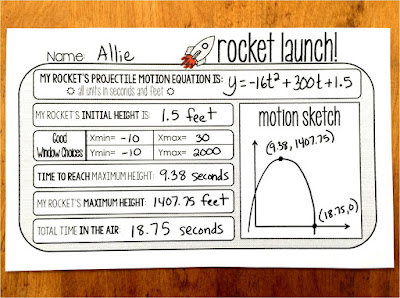## Pages

### Vertex or Zero? Now we know!

Quadratic word problems projectile motion- Scaffolded Math and Science

We've factored. We've used the Quadratic Formula. We've evaluated the discriminant and solved for complex zeros. There was one thing left in our Quadratics unit to cover:

My students' solving abilities are strong and they have become very comfortable with using formulas and plugging things back in to find other values. with word problems, the keywords in the problems are an issue.

I give each student this quadratic keywords poster first (I also have it enlarged in my classroom).

Then, before introducing any word problems with equations, I give this simple quadratic word problems keywords sorting activity that has no equations at all.

The goal is to identify in each scenario if we're being asked to find a zero, the x at the max or the y at the max.free Quadratic word problem keywords sort

By the time I introduce equations, my students are so much more prepared to solve what the word problems are asking them to solve. You can download all of the resources in this post for free by clicking the links of the pictures.projectile motion template

Later when practicing our projectile motion problems, this template gives great practice for finding all of the important information found by graphing quadratics. It also gives students practice finding a good graphing calculator window.